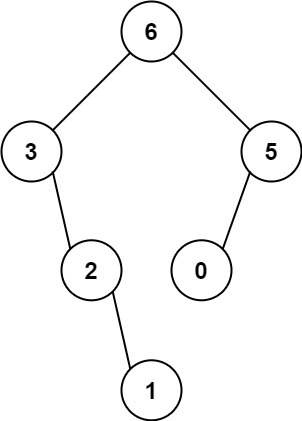# Leetcode 654 - Max binary tree

Note:

• Recursion

You are given an integer array nums with no duplicates. A maximum binary tree can be built recursively from nums using the following algorithm:

• Create a root node whose value is the maximum value in nums.
• Recursively build the left subtree on the subarray prefix to the left of the maximum value.
• Recursively build the right subtree on the subarray suffix to the right of the maximum value.
Return the maximum binary tree built from nums.

Example